# How to Calculate and Solve for Capacity of the Sprinkler System | Irrigation Water Requirement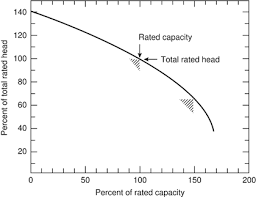The image above represents capacity of the sprinkler system.

To compute for the capacity of the sprinkler system, four essential parameters are needed and these parameters are Area to be Irrigated (A), Net Depth of Water Application (d), Number of Drop allowed for the Completion of One Irrigation (F), Number of Actual Operating Hours per Day (H) and Water Application Efficiency (E).

The formula for calculating capacity of the sprinkler system:

Q = 27800 . A . d / F . H . E

Where:

Q = Capacity of the Sprinkler System
A = Area to be Irrigated
d = Net Depth of Water Application
F = Number of Drop allowed for the Completion of One Irrigation
H = Number of Actual Operating Hours per Day
E = Water Application Efficiency

Let’s solve an example;
Find the capacity of the sprinkler system when the area to be irrigated is 2, the net depth of water application is 8, the number of drop allowed for the completion of one irrigation is 10, the number of actual operating hours per day is 6 and the water application efficiency is 4.

This implies that;

A = Area to be Irrigated = 2
d = Net Depth of Water Application = 8
F = Number of Drop allowed for the Completion of One Irrigation = 10
H = Number of Actual Operating Hours per Day = 6
E = Water Application Efficiency = 4

Q = 27800 . A . d / F . H . E
Q = 27800 . (2) . (8) / (10) . (6) . (4)
Q = 444800 / 240
Q = 1853.3

Therefore, the capacity of the sprinkler system is 1853.3.

Calculating for the Area to be Irrigated when the Capacity of the Sprinkler System, the Net Depth of Water Application, the Number of drop Allowed for the Completion of One Irrgiation, the Number of Actual Operating Hours per Day and the Water Application Efficiency is Given.

A = Q x F . H . E / 27800 . d

Where;

A = Area to be Irrigated
Q = Capacity of the Sprinkler System
d = Net Depth of Water Application
F = Number of Drop allowed for the Completion of One Irrigation
H = Number of Actual Operating Hours per Day
E = Water Application Efficiency

Let’s Solve an example;
Find the area to be irrigated when the capacity of the sprinkler system is 4, the net depth of water application is 6, number of drop allowed for the competion of one irrigation is 2, number of actual operating hours per day is 3 and the water application efficiency is 8.

This implies that;

Q = Capacity of the Sprinkler System = 4
d = Net Depth of Water Application = 6
F = Number of Drop allowed for the Completion of One Irrigation = 2
H = Number of Actual Operating Hours per Day = 3
E = Water Application Efficiency = 8

A = Q x F . H . E / 27800 . d
A = 4 x 2 . 3 . 8 / 27800 . 6
A = 192 / 166800
A = 0.00115

Therefore, the area to be irrigated is 0.00115.

Calculating for the Net Depth of Water Application when the Capacity of the Sprinkler System, the Area to be Irrigated, the Number of drop Allowed for the Completion of One Irrgiation, the Number of Actual Operating Hours per Day and the Water Application Efficiency is Given.

d = Q x F . H . E / 27800 . A

Where;

d = Net Depth of Water Application
Q = Capacity of the Sprinkler System
A = Area to be Irrigated
F = Number of Drop allowed for the Completion of One Irrigation
H = Number of Actual Operating Hours per Day
E = Water Application Efficiency

Let’s Solve an example;
Find the net depth of water application when the capacity of the sprinkler is 8, the area to be irrigated is 2, number of drops allowed for the completion of one irrigation is 4, the number of actual operating hours per day is 9 and the water application efficiency is 7.

This implies that;

Q = Capacity of the Sprinkler System = 8
A = Area to be Irrigated = 2
F = Number of Drop allowed for the Completion of One Irrigation = 4
H = Number of Actual Operating Hours per Day = 9
E = Water Application Efficiency = 7

d = Q x F . H . E / 27800 . A
d = 8 x 4 . 9 . 7 / 27800 . 2
d = 2016 / 55600
d = 0.036

Therefore, the net depth of water application is 0.036.

Calculating for the Number of drop Allowed for the Completion of One Irrgiation when the Capacity of the Sprinkler System, the Net Depth of Water Application, the Area to be Irrigated, the Number of Actual Operating Hours per Day and the Water Application Efficiency is Given.

F = 27800 . A . d / Q x H . E

Where;

F = Number of Drop allowed for the Completion of One Irrigation
Q = Capacity of the Sprinkler System
A = Area to be Irrigated
d = Net Depth of Water Application
H = Number of Actual Operating Hours per Day
E = Water Application Efficiency

Let’s Solve an example;
Find the number of drops allowed for the completion of one irrigation when the capacity of the sprinkler is 12, the area to be irrigated is 3, the net depth of water application is 4, the number of actual operating hours per day is 10 and the water application efficiency is 8.

This implies that;

Q = Capacity of the Sprinkler System = 12
A = Area to be Irrigated = 3
d = Net Depth of Water Application = 4
H = Number of Actual Operating Hours per Day = 10
E = Water Application Efficiency = 8

F = 27800 . A . d / Q x H . E
F = 27800 . 3 . 4 / 12 x 10 . 8
F = 333600 / 960
F = 347.5

Therefore, the number of drops allowed for the completion of one irrigation is 347.5.

Calculating for the Number of Actual Operating Hours per Day when the Capacity of the Sprinkler System, the Net Depth of Water Application, the Area to be Irrigated, the Number of drop Allowed for the Completion of One Irrgiation and the Water Application Efficiency is Given.

H = 27800 . A . d / Q x F . E

Where;

H = Number of Actual Operating Hours per Day
Q = Capacity of the Sprinkler System
A = Area to be Irrigated
d = Net Depth of Water Application
F = Number of Drop allowed for the Completion of One Irrigation
E = Water Application Efficiency

Let’s Solve an example;
Find the number of actual operating hours per day when the capacity of the sprinkler system is 24, the area to be irrigated is 6, the net depth of water application is 8, the number of drop allowed for the completion of one irrigation is 30 and the water application efficiency is 18.

This implies that;

Q = Capacity of the Sprinkler System = 24
A = Area to be Irrigated = 6
d = Net Depth of Water Application = 8
F = Number of Drop allowed for the Completion of One Irrigation = 30
E = Water Application Efficiency = 18

H = 27800 . A . d / Q x F . E
H = 27800 . 6 . 8 / 24 x 30 . 18
H = 1334400 / 12960
H = 102.96

Therefore, the number of actual operating hours per day is 102.96.

Calculating for the Water Application Efficiency when the Capacity of the Sprinkler System, the Net Depth of Water Application, the Area to be Irrigated, the Number of drop Allowed for the Completion of One Irrgiation and the Number of Actual Operating Hours per Day is Given.

E = 27800 . A . d / Q x F . H

Where;

E = Water Application Efficiency
Q = Capacity of the Sprinkler System
A = Area to be Irrigated
d = Net Depth of Water Application
F = Number of Drop allowed for the Completion of One Irrigation
H = Number of Actual Operating Hours per Day

Let’s Solve an example;
Find the water application efficiency when the capacity of the sprinkler system is 32, the area to be irrigated is 5, the net depth of water application is 2, the number of drop allowed for the completion of one irrigation is 28 and the number of actual operating hours per day is 22.

This implies that;

Q = Capacity of the Sprinkler System = 32
A = Area to be Irrigated = 5
d = Net Depth of Water Application = 2
F = Number of Drop allowed for the Completion of One Irrigation = 28
H = Number of Actual Operating Hours per Day = 22

E = 27800 . A . d / Q x F . H
E = 27800 . 5 . 2 / 32 x 28 . 22
E = 278000 / 19712
E = 14.10

Therefore, the water application efficiency is 14.10.

Nickzom Calculator – The Calculator Encyclopedia is capable of calculating the capacity of the sprinkler system.

To get the answer and workings of the capacity of the sprinkler system using the Nickzom Calculator – The Calculator Encyclopedia. First, you need to obtain the app.

You can get this app via any of these means:

To get access to the professional version via web, you need to register and subscribe for NGN 2,000 per annum to have utter access to all functionalities.
You can also try the demo version via https://www.nickzom.org/calculator

Apple (Paid) – https://itunes.apple.com/us/app/nickzom-calculator/id1331162702?mt=8
Once, you have obtained the calculator encyclopedia app, proceed to the Calculator Map, then click on Agricultural under Engineering.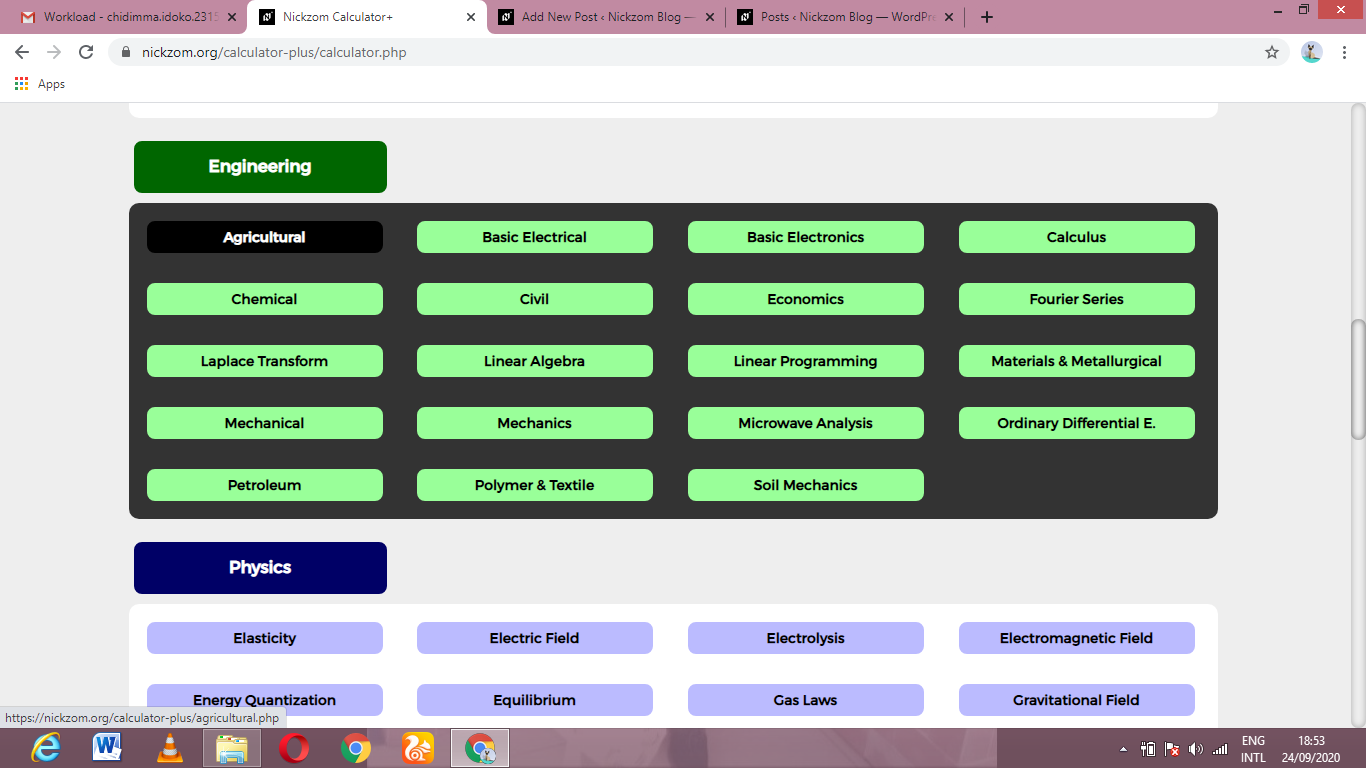Now, Click on Irrigation Water Requirement under Agricultural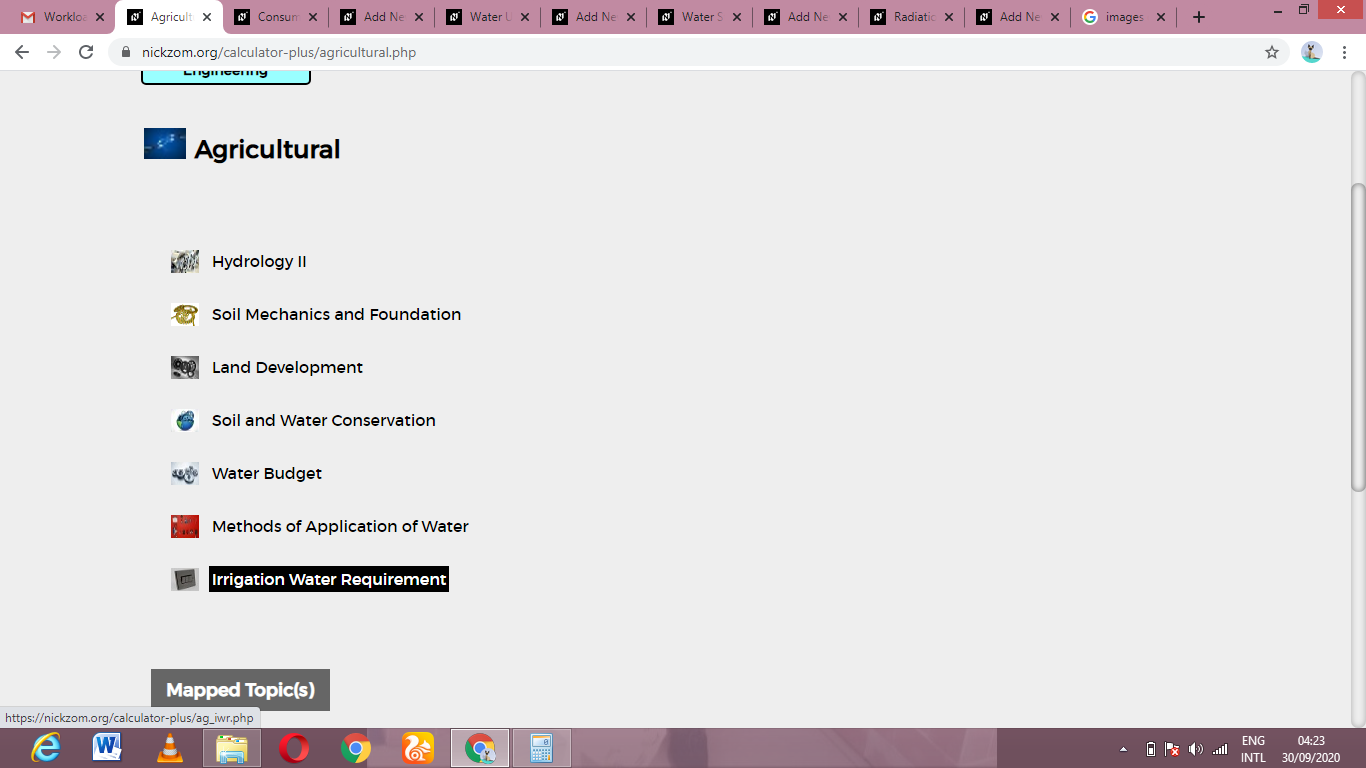Now, Click on Capacity of the Sprinkler System under Irrigation Water Requirement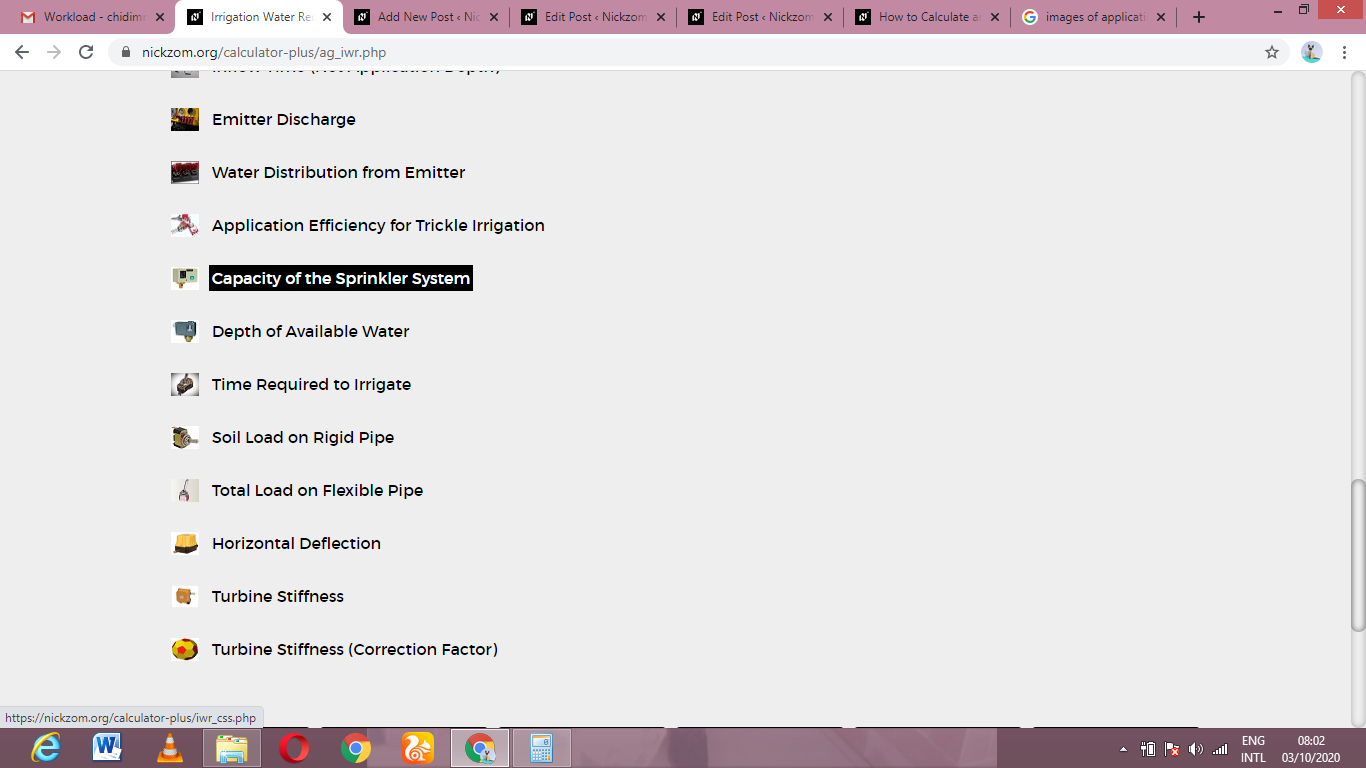The screenshot below displays the page or activity to enter your values, to get the answer for the capacity of the sprinkler system according to the respective parameters which is the Area to be Irrigated (A), Net Depth of Water Application (d), Number of Drop allowed for the Completion of One Irrigation (F), Number of Actual Operating Hours per Day (H) and Water Application Efficiency (E).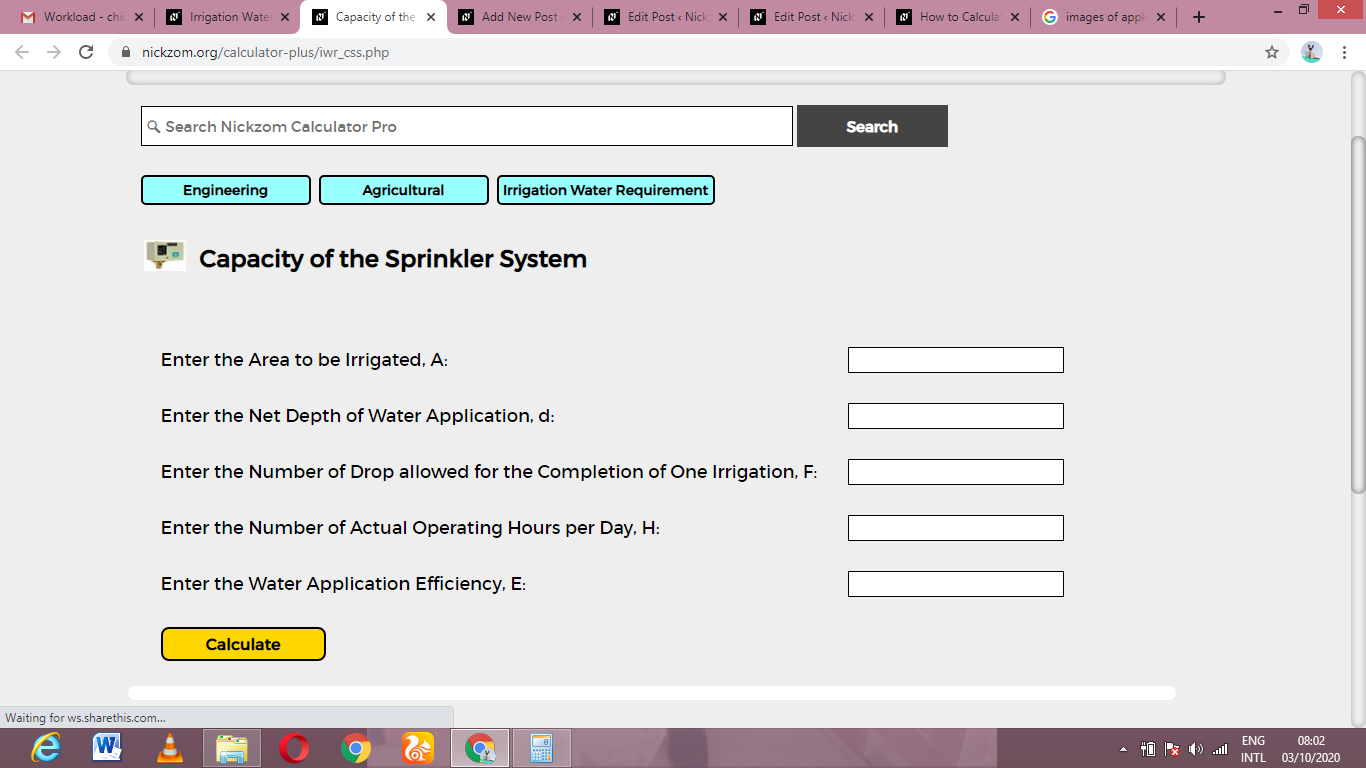Now, enter the values appropriately and accordingly for the parameters as required by the Area to be Irrigated (A) is 2, Net Depth of Water Application (d) is 8, Number of Drop allowed for the Completion of One Irrigation (F) is 10, Number of Actual Operating Hours per Day (H) is 6 and Water Application Efficiency (E) is 4.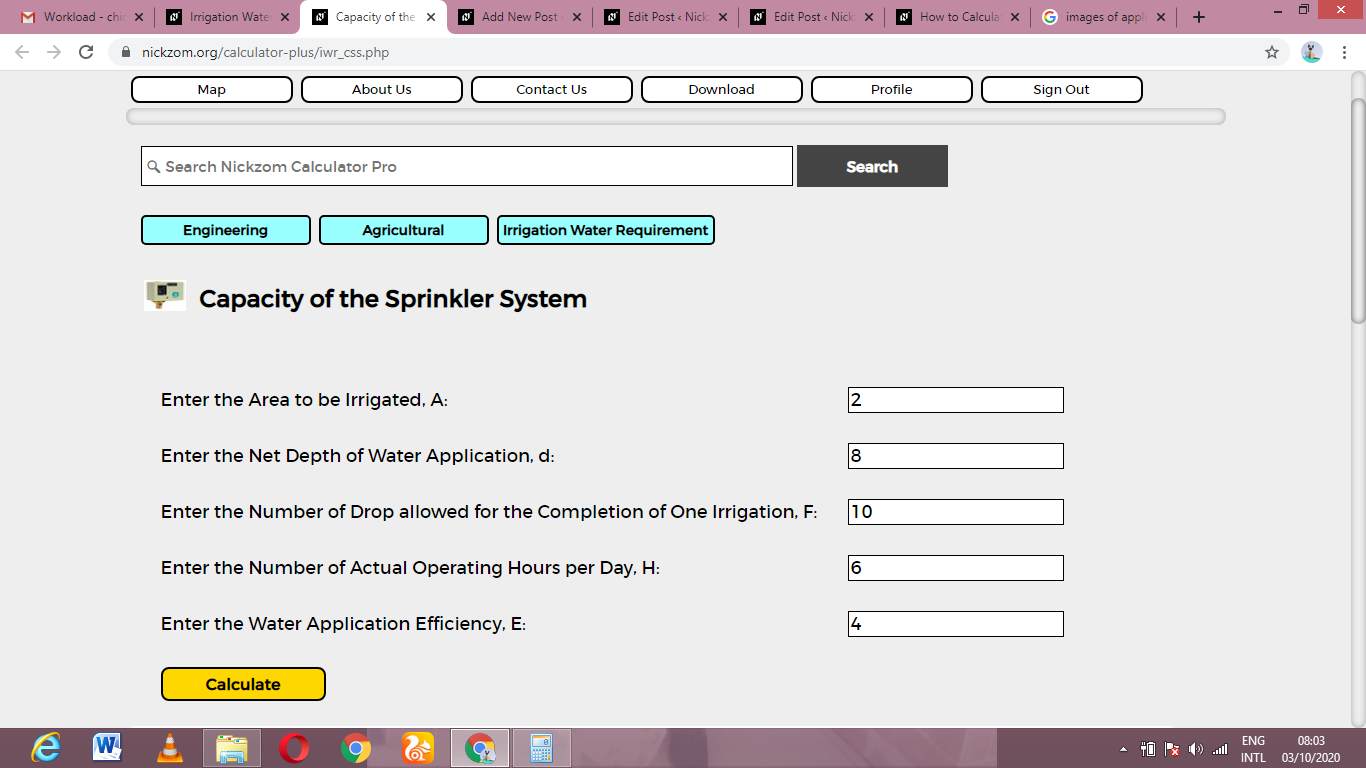Finally, Click on Calculate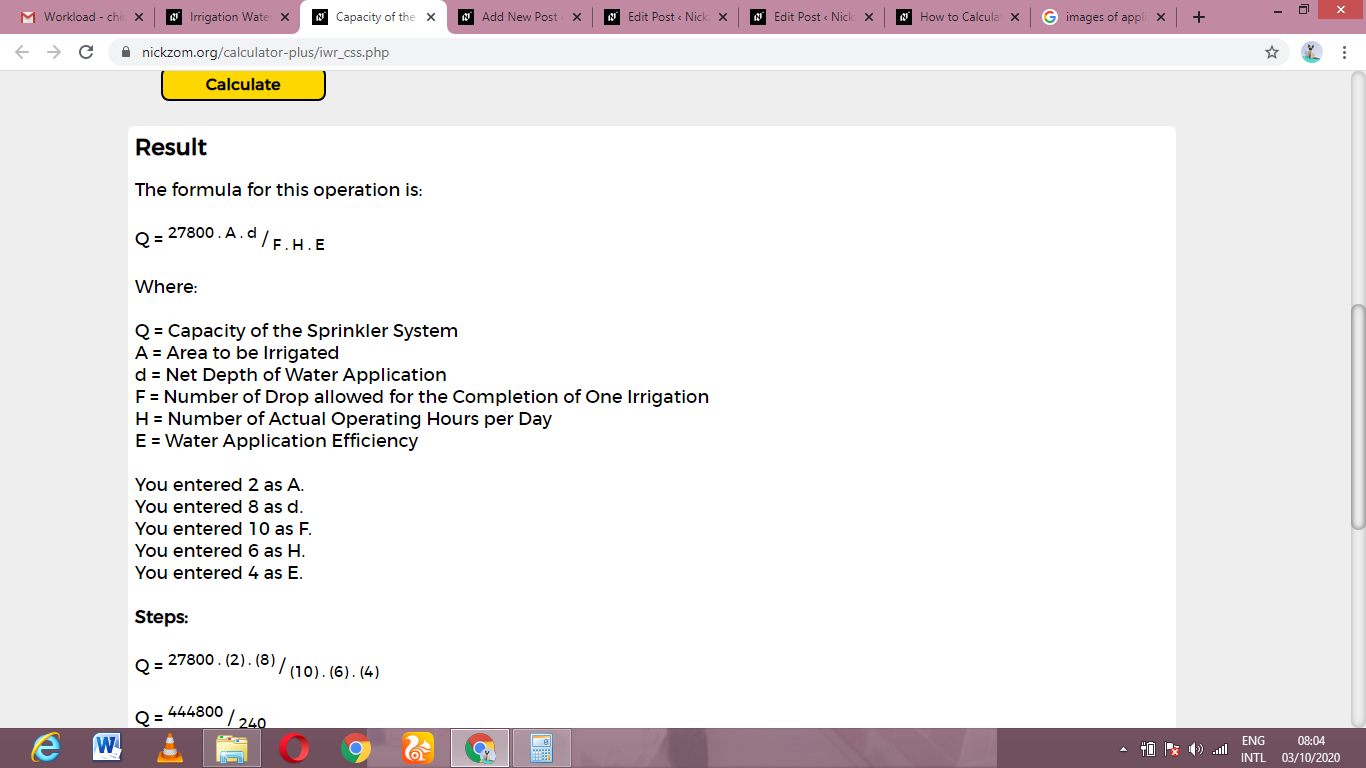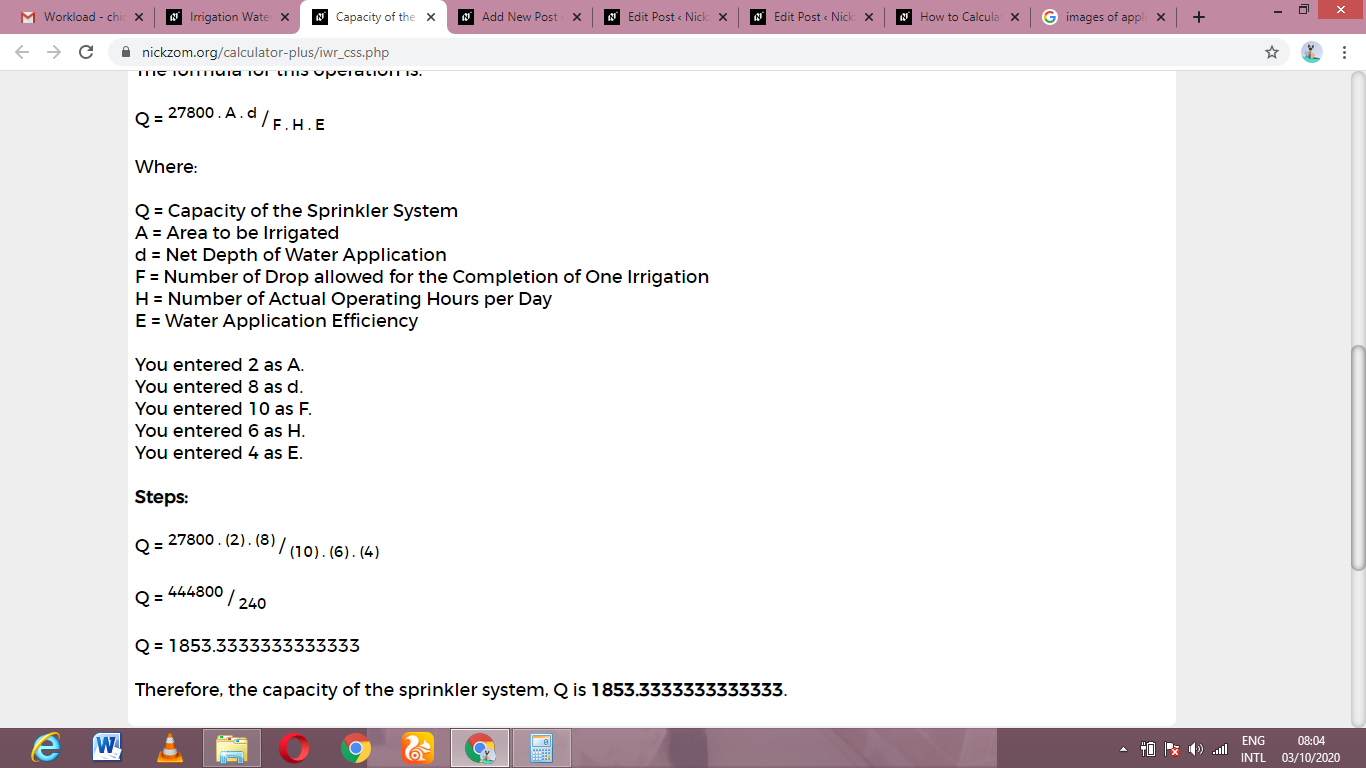As you can see from the screenshot above, Nickzom Calculator– The Calculator Encyclopedia solves for the capacity of the sprinkler system and presents the formula, workings and steps too.